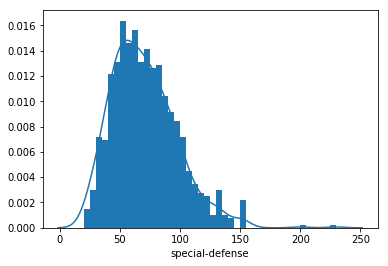Python：matplotlib 之直方图 (二十八)

直方图

plt.hist(data = df, x = 'num_var')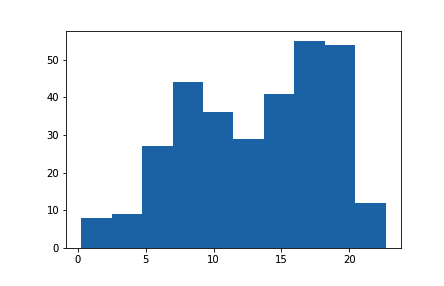bin_edges = np.arange(0, df['num_var'].max()+1, 1)
plt.hist(data = df, x = 'num_var', bins = bin_edges)

arange 的第一个参数是最左侧的分箱边缘，第二个参数是上限，第三个参数是分箱宽度。注意，即使我在第二个参数中指定了“max”值，我添加了“+1”（分箱宽度）。这是因为 arange 将仅返回完全小于上限的值。加上“+1”可有效地确保左右侧的分箱边缘至少是最大数据值，以便所有数据点都能绘制出来。最左侧的分箱设为硬编码的值，以便获得可解释的值，当然你也可以使用 numpy 的 around 等函数以程序方式达到这种效果。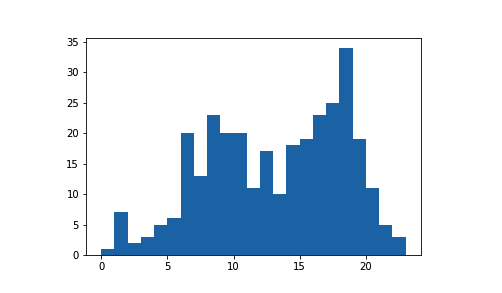plt.figure(figsize = [10, 5]) # larger figure size for subplots

# histogram on left, example of too-large bin size
plt.subplot(1, 2, 1) # 1 row, 2 cols, subplot 1
bin_edges = np.arange(0, df['num_var'].max()+4, 4)
plt.hist(data = df, x = 'num_var', bins = bin_edges)

# histogram on right, example of too-small bin size
plt.subplot(1, 2, 2) # 1 row, 2 cols, subplot 2
bin_edges = np.arange(0, df['num_var'].max()+1/4, 1/4)
plt.hist(data = df, x = 'num_var', bins = bin_edges)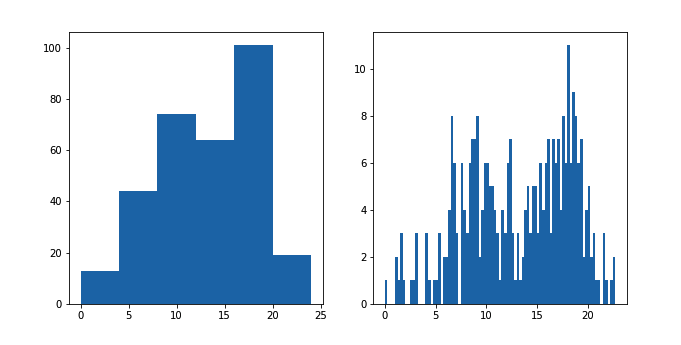替代方法

seaborn 函数 distplot也可以用于绘制直方图，并且与其他单变量绘图函数集成到一起。

sb.distplot(df['num_var'])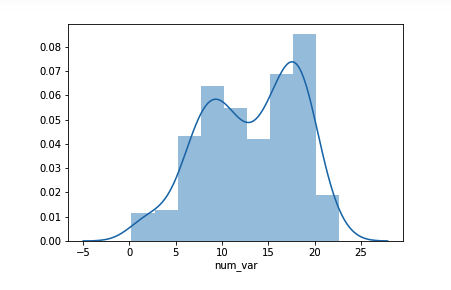distplot 函数具有指定直方图分箱的内置规则，默认情况下，会在数据上方绘制一个 (KDE) 核密度估计。纵轴基于 KDE，而不是直方图：长条的高度之和不一定等于 1，但是曲线下方的面积应该等于 1。如果你想详细了解 KDE，请参阅这节课末尾的补充内容。

bin_edges = np.arange(0, df['num_var'].max()+1, 1)
sb.distplot(df['num_var'], bins = bin_edges, kde = False,
hist_kws = {'alpha' : 1})

alpha（透明度）设置必须当做字典与 "hist_kws" 关联，因为还有其他底层绘图函数（例如 KDE）具有自己的可选关键字参数。直方图练习

# prerequisite package imports
import numpy as np
import pandas as pd
import matplotlib.pyplot as plt
import seaborn as sb

%matplotlib inline

from solutions_univ import histogram_solution_1

pokemon = pd.read_csv('./data/pokemon.csv')
pokemon.head()
id species generation_id height weight base_experience type_1 type_2 hp attack defense speed special-attack special-defense
0 1 bulbasaur 1 0.7 6.9 64 grass poison 45 49 49 45 65 65
1 2 ivysaur 1 1.0 13.0 142 grass poison 60 62 63 60 80 80
2 3 venusaur 1 2.0 100.0 236 grass poison 80 82 83 80 100 100
3 4 charmander 1 0.6 8.5 62 fire NaN 39 52 43 65 60 50
4 5 charmeleon 1 1.1 19.0 142 fire NaN 58 64 58 80 80 65

# YOUR CODE HERE
# 1.简单的直方图
# plt.hist(data=pokemon, x='special-defense')

# 2.使用 numpy 的 arange 函数设置这些分箱边缘
bin_edges = np.arange(0, pokemon['special-defense'].max() + 10, 10)
print(bin_edges)
plt.hist(data=pokemon, x='special-defense', bins=bin_edges)
[  0  10  20  30  40  50  60  70  80  90 100 110 120 130 140 150 160 170
180 190 200 210 220 230]

(array([   0.,    0.,   18.,   57.,  102.,  125.,  116.,  108.,   94.,
71.,   47.,   25.,   14.,   16.,    3.,    9.,    0.,    0.,
0.,    0.,    1.,    0.,    1.]),
array([  0,  10,  20,  30,  40,  50,  60,  70,  80,  90, 100, 110, 120,
130, 140, 150, 160, 170, 180, 190, 200, 210, 220, 230]),
<a list of 23 Patch objects>)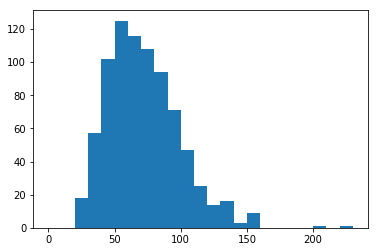# run this cell to check your work against ours
histogram_solution_1()

I've used matplotlib's hist function to plot the data. I have also used numpy's arange function to set the bin edges. A bin size of 5 hits the main cut points, revealing a smooth, but skewed curves. Are there similar characteristics among Pokemon with the highest special defenses?bin_edges = np.arange(0, pokemon['special-defense'].max()+5, 5)
sb.distplot(pokemon['special-defense'], bins = bin_edges, kde = True,
hist_kws = {'alpha' : 1})
<matplotlib.axes._subplots.AxesSubplot at 0x7f3f8536bdd8>